CBSE Sample Papers for Class 10 SA2 Maths Solved 2016 Set 13

# CBSE Sample Papers for Class 10 SA2 Maths Solved 2016 Set 13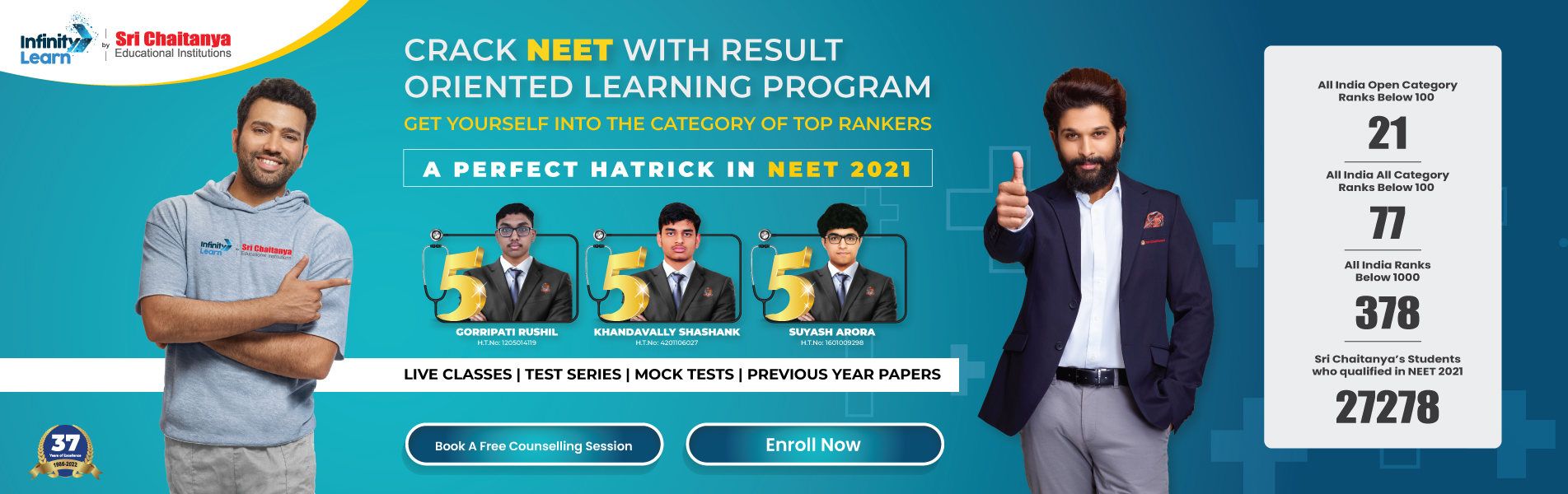Infinity Learn NEET IIT-JEE
Infinity Learn NEET
banner-chaitanya-web

SECTION A

1.The sum of the ages of two friends is 20. Four years ago, the product of their ages in years was 48. Is it possible to determine their present ages?

Free IIT-JEE/NEET/NCERT/CBSE Material

Download 100,000+ FREE PDFs, solved questions, Previous Year Papers, Quizzes, and Puzzles directly to your Whatsapp account!
3. In the given figure, AB, AC and AD are tangents to the circles with centre O and O’. If AD = 10 cm, find AB
4.The probability of getting a defective pen from a lot of 500 pens is 1/25 Find the number of defective pensin the lot.

SECTION B
5.For what value of k, given equation has real and equal roots: (k – l)x2 – 2(k – l)x +1 = 0?

6.In the given figure, there are two concentric circles with centre O and of radii 5 cm and 3 cm. From an external point P, tangents PA and PB are drawn to these circles. If AP = 12 cm, find the length of BP
7.Which term of the Arithmetic Progression 3, 10, 17,… will be 84 more than its 13th term?

8.Prove that tangents drawn at the ends of a diameter of a circle are parallel.

9.Find the value of k, if the distance between the points (2, k) and (4, 3) is 8.

10.Find the coordinates of a point P which lies on the line segment joining the points A (-2, 0) and B(0, 8)such that AP = 1/4 AB.

SECTION C
12.A tree breaks due to storm and the broken part bends so that the top of the tree touches the ground making an angle 30° with it. The distance between the foot of the tree to the point where the top touches the ground is 8 m. Find the height of the tree.

13.On the first day of the strike of doctors in a hospital, the attendance at its OPD was 1040 patients. As the strike continues, the attendance declined by 80 patients every day. Find from which day of the strike the OPD would have no patient.

14.A juice seller serves his customers using a glass as shown in figure. The inner diameter of the cylindrical glass is 5 cm, but the bottom of the glass has a hemispherical raised portion which reduces the capacity of the glass. If the height of the glass is 10 cm, find the apparent capacity of the glass and its actual capacity. (Use n = 3.14)
16.A solid is in the form of a right circular cone mounted on a hemisphere. The radius of the hemisphere is 4.2 cm and height of the cone is 8 cm. The solid is placed in a cylindrical tub full of water in such a way that whole solid is submerged in the water. If the radius of the cylinder is 10 cm and its height is 19.6 cm, find the volume of water left in the cylindrical tub. (Use pi =22/7)

17.An equilateral triangle has two vertices at the points (3, 4) and (- 2, 3). Find the coordinates of the third vertex.

18.A hemispherical bowl of internal radius of 30 cm contains a liquid. The liquid is to be filled into cylindrical shaped bottles of diameter 10 cm and height 12 cm. How many bottles are necessary to empty the bowl, if 10% of the liquid is wasted during the process of filling the bottles?

19.The diameter of internal and external surfaces of a hollow spherical vessel are 6 cm and 10 cm respectively.If it is melted and recast into a solid cylinder of height 2~ cm, find the diameter of the cylinder.

20.In the given figure, the shape of the top of a table in a restaurant is that of a sector of a circle with centre O and BOD = 90°, if BO = OD = 60 cm find :
(i)the area of the top of the table
(ii)the perimeter of the table top. (Take pi =3.14)
SECTION D

21.Students of class ‘X’ collected Rs 9000. They wanted to divide it equally among a certain number of students residing in slum areas. When they started distributing the amount, 20 more students from nearby slum also joined. Now each student got Rs 160 less.
(a)Find the original number of students living in the slum.
(b)Which value is depicted by students of class X?

22.A spiral is made up of successive semicircles, with centres alternately at A and B, starting with centre at A, of radii 0.5 cm, 1.0 cm, 1.5 cm, 2.0 cm, … . What is the total length of such a spiral made up of thirteen consecutive semicircle?(taken pi=22/7)
23.O is the centre of a circle. PA and PB are tangents to the circle from a point P. Prove that (i) PAOB is a cyclic quadrilateral (ii) PO is the bisector of angle APB (iii) angle OAB = angle OPA.

24.QR is a tangent at Q. PR || AQ, where AQ is a chord through A and P is the centre of the circle with the end point of the diameter AB. Prove that BR is tangent at B.
25.Cards numbered 2, 3, 4, 26 are put in a box and mixed thoroughly. One person draws a card from the box. Find the probability that number on the card is (i) even (ii) prime (iii) a perfect square (iv) divisible by 2 or 5.

26.The angle of elevation of a cloud from a point 200 m above a lake is 30° and the angle of depression of its reflection in the lake is 60°. Find the height of the cloud above the lake.

27.Draw a right triangle in which the sides (other than hypotenuse) are of lengths 6 cm and 8 cm. Then construct another triangle whose sides are 4/3 times the corresponding sides of the given triangle.

28.A(6, 1), B(8, 2) and C(9, 4) are three vertices of a parallelogram ABCD. If E is the mid-point of DC, find the area of triangle ADE.
30.A tent is in the shape of a cylinder surmounted by a conical top. If the height and diameter of the cylindrical part are 2.1 m and 4 m respectively and the slant height of the top is 2.8 m, find the area of the canvas used for making the tent. Find the cost of the canvas of the tent at the rate of Rs 500 per m2. Also, find the volume of air enclosed in the tent.

31.The area of an equilateral triangle is 17320.5 cm2. With each vertex of the triangle as centre, a circle is described with radius equal to half the length of the side of the triangle. Find the area of the triangle not included in the circle.

Join Infinity Learn Regular Class Programme!